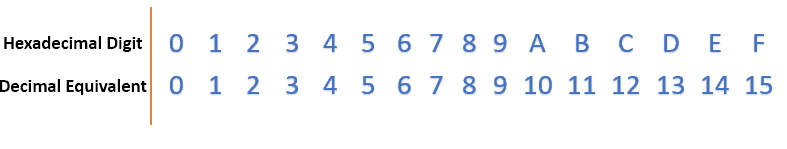### Get started with Spring and Spring Boot, through the Learn Spring course:

>> CHECK OUT THE COURSE

## 1. Introduction

Converting a hexadecimal (Hex) string into an integer is a frequent task during programming, particularly when handling data types that use hexadecimal notations.

In this tutorial, we’ll dive into various approaches to converting a Hex String into an int in Java.

Hexadecimal employs base-16, resulting in each digit that could take on 16 possible values from zero through nine, followed by (A) through (F):Let’s also note that in most cases, hexadecimal strings begin with “0x” to denote its base.

## 3. Using Integer.parseInt()

The easiest way of converting a hex string to an integer in Java is via the Integer.parseInt() method. It converts a string into an integer, assuming the base in which it was written. For us, the base is 16:

``````@Test
public void givenValidHexString_whenUsingParseInt_thenExpectCorrectDecimalValue() {
String hexString = "0x00FF00";
int expectedDecimalValue = 65280;

int decimalValue = Integer.parseInt(hexString.substring(<span class="hljs-number">2</span>), 16);

assertEquals(expectedDecimalValue, decimalValue);
}``````

In the above code, the hexadecimal string “0x00FF00” is converted to its corresponding decimal value of 65280 using Integer.parseInt, and the test asserts that the result matches the expected decimal value. Note that we use the substring(2) method to remove the “ox” part from the hexString.

## 4. Using BigInteger

For more flexibility when working with very large or unsigned hexadecimal values, we can consider using a BigInteger. It operates on arbitrary precision integers and can, therefore, be used in myriad contexts.

Here’s how we can convert a hex string to a BigInteger and then extract the integer value:

``````@Test
public void givenValidHexString_whenUsingBigInteger_thenExpectCorrectDecimalValue() {
String hexString = "0x00FF00";
int expectedDecimalValue = 65280;

BigInteger bigIntegerValue = new BigInteger(hexString.substring(2), 16);
int decimalValue = bigIntegerValue.intValue();
assertEquals(expectedDecimalValue, decimalValue);
}``````

## 5. Using Integer.decode()

Another way for changing a Hex string into an integer is provided by the Integer.decode() method. This approach deals with hexadecimal as well as decimal strings.

Here, we use Integer.decode() without stating the base as it is determined from a string itself:

``````@Test
public void givenValidHexString_whenUsingIntegerDecode_thenExpectCorrectDecimalValue() {
String hexString = "0x00FF00";
int expectedDecimalValue = 65280;

int decimalValue = Integer.decode(hexString);

assertEquals(expectedDecimalValue, decimalValue);
}``````

Because the Integer.decode() method can handle the “0x” prefix in the string, we don’t need to manually remove it using substring(2) as we did in the previous approaches.

## 6. Conclusion

In conclusion, we discussed the significance of hexadecimal representation and delved into three distinct approaches: Integer.parseInt() for a straightforward conversion, BigInteger for handling large or unsigned values, and Integer.decode() for versatility in handling both hexadecimal and decimal strings, including the “0x” prefix.

As always, the complete code samples for this article can be found over on GitHub.

### Get started with Spring and Spring Boot, through the Learn Spring course:

>> CHECK OUT THE COURSE
Subscribe
Notify of